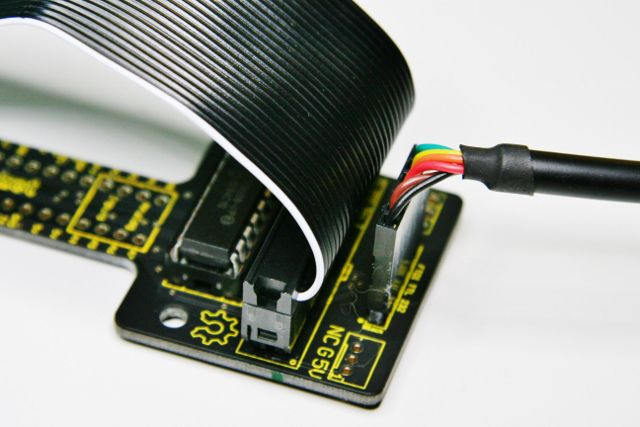Before you start, ensure that the SPI driver is loaded on your Raspberry Pi distribution.

The following Python sample reads from a TMP36 analog temperature sensor every second. It converts the raw temperature data into millivolts, degrees Celsius and Fahrenheit. For reliable and efficient SPI communication (no bit-banging), the sample depends on the py-spidev Python module. To install py-spidev, do the following, assuming that the 'git' and 'python-dev' packages are already installed on the Raspberry Pi:

git clone https://github.com/doceme/py-spidev.git cd /py-spidev python setup.py install

Then, using the 'nano' editor, do the following:

nano tmp36.py

Paste the sample code below into the editor, save it and exit.

Then, make tmp36.py executable and run it.

chmod +x tmp36.py ./tmp36.py

The output of the script should look like this:

raw temp= 206 mV= 663.8671875 Celsius= 16.38671875 Fahrenheit= 61.49609375

Yeah, my office is a tad nippy at the moment ;)

#!/usr/bin/python

import spidev
import time

spi = spidev.SpiDev()
spi.open(0,0)

# read SPI data from one of the MCP3008's eight possible inputs (0 thru 7)
return -1
adcout = ((r&3) << 8) + r

# TMP36 temperature sensor connected to ADC input 0
TempSensor = 0

while True:
# Convert the raw ADC input to milliVolts, degrees Celsius and Fahrenheit
milliVolts = rawTemp * (3300.0 / 1024.0)
tempCelsius = ((milliVolts - 100.0) / 10.0) - 40.0
tempFahrenheit = (tempCelsius * 9.0 / 5.0 ) + 32

print "raw temp=", rawTemp
print "mV=", milliVolts
print "Celsius=", tempCelsius
print "Fahrenheit=", tempFahrenheit

time.sleep(1)

Original author: Fabien Royer, nwazet.com